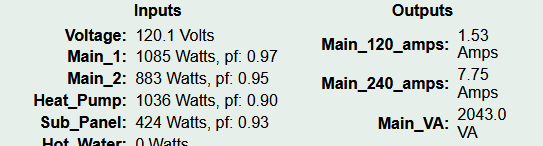# Calcuating Amps on a 4 wire 220v circuit

When calculating amps on a double pole 220v breaker, is there an easy way to get a correct value? For example, for my stove I have a double pole 220v breaker that I have CT’s on both hots. One hot wire also feeds the 120v electronics of the stove (along with the neutral). So, when the stove is idle, the one leg reads 13 watts and the other is 0 watts. But, when it is in use, the 13w is 120v and the rest is 220 which results in a wrong calculation on one leg. I can just divide the watts by 220v on the second leg by 2 to get the amps, but for the first leg, the 13 watts needs to be divided by 120 and the rest needs to be divided by 220 and then divided by 2.

Basically, I am asking if there is a way for me to connect the CT’s to get the correct amps with mixed 120/220 volts on a breaker as in an appliance that uses both on one breaker. Do i need a new CT for the neutral wire to calculate?

Thanks

Now let me unpack the question.

With split-phase, if one leg is 120V, the combined voltage is 240V. So I’m going to use those metrics because they add up correctly.

A CT measures the current in a conductor, regardless of the voltage. Current is current. When you place a CT on each of the two conductors of a three-wire appliance, you are measuring the true RMS current in each of the conductors. If the two CTs measure the same, there will be no current on the neutral wire. If they are different, the difference will be carried on the neutral conductor. The current into and out of the stove is always equal, regardless of which of the three conductors are carrying it.

Without getting into what might be happening inside the stove, it’s safe to conceptualize that the current on the lower of the two CTs is being used at 240V and the difference between the two CTs is being used at 120V.

So if you were to label your two stove CTs as say stove_1 and stove_2, you could define the outputs with units=Amps:

`stove_240_amps = stove_1 min stove_2`
`stove_120_amps = (stove_1 - stove_2) abs`

Lets look at an example. All of my 240V circuits use one CT - except my mains. So lets go with that. My mains are Main-1 and Main-2. So I can define:Taking a snapshot of that I get:Taking
`Main_240_amps x voltage x voltage`
I get 7.75 x 120.1 x 120.1 = 1861
`Main_120_amps x voltage`
I get 1.53 x 120.1 = 184
1861 + 184 = 2045, which matches the Main_VA.

Thank you very much for the excellent detailed information. I think I set it up correctly.

I wanted total 120/240 amps of the whole panel and total 120v240 amps of devices connected to the IoTaWatt. For my two other IoTaWatt units, I will get the total 120/240 amps of the units. (I didn’t set them up for the amps calculations yet.) The three units are covering every circuit in the panel box.

Here is my first unit covering the main breakers and circuits 1 to 12.

Did I make any obvious mistakes in the formulas? Thank you again for your help.

PS: I wanted all circuits covered because I integrated the IoTaWatt with my home automation device (Hubitat Elevation). This enables me to have so much control over the systems in my home. For example, If my radon fan unit starts to run erratically or stops, it will send an email to me. Endless possibilities!

Minor question/clarification - where you show:

7.75 x 120.1 x 120.1 = 1861
I think you mean this:
7.75 x 120.1 x 2 or 7.75 x (120.1 + 120.1)

right?